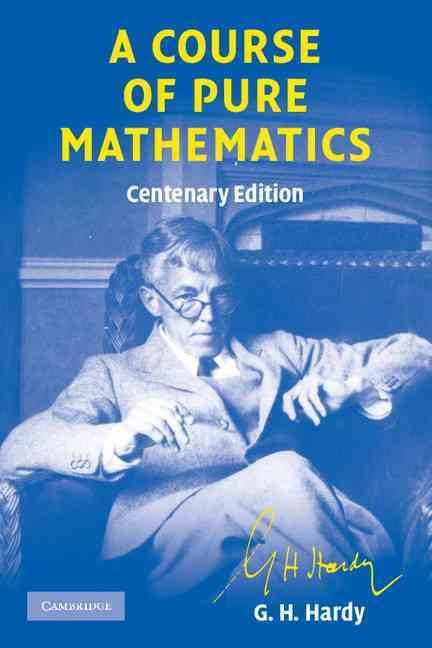# A Course of Pure Mathematics

Updated on
Covid-19
8/101 Votes Alchetron
8
1 Ratings
 10 0 9 0 8 1 7 0 6 0 5 0 4 0 3 0 2 0 1 0
Rate This

Rate This

Country  England
Publication date  1908
Author
Subject  Mathematical analysis

Language  English
Originally published  1908
ISBN  0521720559Publisher  Cambridge University Press
Similar  Works by G H Hardy, Mathematics books, Calculus books

A Course of Pure Mathematics is a classic textbook in introductory mathematical analysis, written by G. H. Hardy. It is recommended for people studying calculus. First published in 1908, it went through ten editions (up to 1952) and several reprints. It is now out of copyright in UK and is downloadable from various internet web sites. It remains one of the most popular books on pure mathematics.

## Contents

The book contains a large number of descriptive and study materials together with a number of difficult problems with regards to number theory analysis. The book is organized into the following chapters, with each chapter further divided.

I. REAL VARIABLES

II. FUNCTIONS OF REAL VARIABLES

III COMPLEX NUMBERS

IV LIMITS OF FUNCTIONS OF A POSITIVE INTEGRAL VARIABLE

V LIMITS OF FUNCTIONS OF A CONTINUOUS VARIABLE. CONTINUOUS AND DISCONTINUOUS FUNCTIONS

VI DERIVATIVES AND INTEGRALS

VII ADDITIONAL THEOREMS IN THE DIFFERENTIAL AND INTEGRAL CALCULUS

VIII THE CONVERGENCE OF INFINITE SERIES AND INFINITE INTEGRALS

IX THE LOGARITHMIC, EXPONENTIAL AND CIRCULAR FUNCTIONS OF A REAL VARIABLE

X THE GENERAL THEORY OF THE LOGARITHMIC, EXPONENTIAL AND CIRCULAR FUNCTIONS

Appendices

INDEX

## Review

The book was intended to help reform mathematics teaching in the UK, and more specifically in the University of Cambridge and in schools preparing to study higher mathematics. It was aimed directly at "scholarship level" students – the top 10% to 20% by ability. Hardy himself did not originally find a passion for mathematics, only seeing it as a way to beat other students, which he did decisively, and gain scholarships. However, his book excels in effectively explaining analytical number theory and calculus following the rigor of mathematics.

Whilst his book changed the way the subject was taught at university, the content reflects the era in which the book was written. The whole book explores number theory and the author constructs real numbers theoretically. It adequately deals with single-variable calculus, sequences, number series, properties of cos, sin, log, etc. but does not refer to mathematical groups, multi-variable functions or vector calculus. Each section includes some demanding problems. Hardy combines the enthusiasm of the missionary with the rigor of the purist in his exposition of the fundamental ideas of the differential and integral calculus, of the properties of infinite series and of other topics involving the notion of limit. Hardy's presentation of mathematical analysis is as valid today as when first written: students will find that his economical and energetic style of presentation is one that modern authors rarely come close to. Despite its limitations, it is considered a classic in its field. It is probably of most use to 1st year university students of pure mathematics.

## References

Topics

 B i Link H2
 L